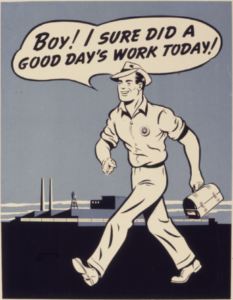## Applications with Rational Equations

### Learning Outcomes

• Solve work problems
• Define and solve an equation that represents the concentration of a mixture

## Rational Formulas

Rational formulas can be useful tools for representing real-life situations and for finding answers to real problems. Equations representing direct, inverse, and joint variation are examples of rational formulas that can model many real-life situations. As you will see, if you can find a formula, you can usually make sense of a situation.

When solving problems using rational formulas, it is often helpful to first solve the formula for the specified variable. For example, work problems ask you to calculate how long it will take different people working at different speeds to finish a task. The algebraic models of such situations often involve rational equations derived from the work formula, $W=rt$. The amount of work done (W) is the product of the rate of work (r) and the time spent working (t). Using algebra, you can write the work formula $3$ ways:

$W=rt$

Find the time (t): $t=\frac{W}{r}$ (divide both sides by r)

Find the rate (r): $r=\frac{W}{t}$(divide both sides by t)

### Example

The formula for finding the density of an object is $D=\frac{m}{v}$, where D is the density, m is the mass of the object, and v is the volume of the object. Rearrange the formula to solve for the mass (m) and then for the volume (v).

Now let us look at an example using the formula for the volume of a cylinder.

### Example

The formula for finding the volume of a cylinder is $V=\pi{r^{2}}h$, where V is the volume, r is the radius, and h is the height of the cylinder. Rearrange the formula to solve for the height (h).

In the following video, we give another example of solving for a variable in a formula, or as they are also called, a literal equation.

## Work

Rational equations can be used to solve a variety of problems that involve rates, times, and work. Using rational expressions and equations can help you answer questions about how to combine workers or machines to complete a job on schedule.Finished after a good day’s work.

A “work problem” is an example of a real life situation that can be modeled and solved using a rational equation. Work problems often ask you to calculate how long it will take different people working at different speeds to finish a task. The algebraic models of such situations often involve rational equations derived from the work formula, $W=rt$. (Notice that the work formula is very similar to the relationship between distance, rate, and time, or $d=rt$.) The amount of work done (W) is the product of the rate of work (r) and the time spent working (t). The work formula has $3$ versions.

$\begin{array}{l}W=rt\\\\\,\,\,\,\,t=\frac{W}{r}\\\\\,\,\,\,\,r=\frac{W}{t}\end{array}$

Some work problems include multiple machines or people working on a project together for the same amount of time but at different rates. In that case, you can add their individual work rates together to get a total work rate. Let us look at an example.

### Example

Myra takes $2$ hours to plant $50$ flower bulbs. Francis takes $3$ hours to plant $45$ flower bulbs. Working together, how long should it take them to plant $150$ bulbs?

Other work problems go the other way. You can calculate how long it will take one person to do a job alone when you know how long it takes people working together to complete the job.

### Example

Joe and John are planning to paint a house together. John thinks that if he worked alone, it would take him $3$ times as long as it would take Joe to paint the entire house. Working together, they can complete the job in $24$ hours. How long would it take each of them, working alone, to complete the job?

In the video that follows, we show another example of finding one person’s work rate given a combined work rate.

As shown above, many work problems can be represented by the equation $\frac{t}{a}+\frac{t}{b}=1$, where t is the time to do the job together, a is the time it takes person A to do the job, and b is the time it takes person B to do the job. The $1$ refers to the total work done—in this case, the work was to paint $1$ house.

The key idea here is to figure out each worker’s individual rate of work. Then, once those rates are identified, add them together, multiply by the time t, set it equal to the amount of work done, and solve the rational equation.

### Try It

We present another example of two people painting at different rates in the following video.

## Mixing

Mixtures are made of ratios of different substances that may include chemicals, foods, water, or gases. There are many different situations where mixtures may occur both in nature and as a means to produce a desired product or outcome. For example, chemical spills, manufacturing, and even biochemical reactions involve mixtures. The thing that can make mixtures interesting mathematically is when components of the mixture are added at different rates and concentrations. In our final example, we will define an equation that models the concentration  – or ratio of sugar to water – in a large mixing tank over time. You are asked whether the final concentration of sugar is greater than the concentration at the beginning.

### Example

A large mixing tank currently contains $100$ gallons of water into which $5$ pounds of sugar have been mixed. A tap will open pouring $10$ gallons per minute of water into the tank at the same time sugar is poured into the tank at a rate of $1$ pound per minute. Find the concentration (pounds per gallon) of sugar in the tank after $12$ minutes. Is that a greater concentration than at the beginning?

In the following video, we show another example of how to use rational functions to model mixing.

## Contribute!

Did you have an idea for improving this content? We’d love your input.